# Using Matrices to Generate the Fibonacci Sequence

By Alex Beal
April 10, 2011

Here’s a fun little matrix: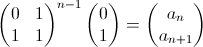That generates the an and an+1 terms of the Fibonacci sequence.1 To see why, let’s look at a recursive definition of the Fibonacci sequence.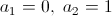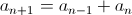That’s easy enough to understand. The first and second terms are both 1, and the next term is the sum of the last term plus the current term. To see how this relates to the matrix above, we must turn this into a matrix equation. The trick here is to have a good understanding of matrix multiplication. If you need a refresher, here’s a site with a tutorial. Let’s look at an example of a 2x2 matrix multiplied by a 2x1 (even if you have a good understanding of matrix multiplication, hang tight, this is going somewhere).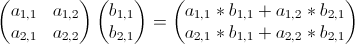Now let’s make some substitutions and multiply it out: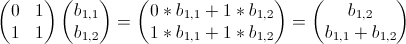As you can see, the effect of multiplying the b matrix by the Fibonacci matrix is that it moves the b1,2 position to the b1,1 position, and the b1,2 gets filled with the sum of b1,1 and b1,2. Let’s now substitute the b’s for an-1 and an: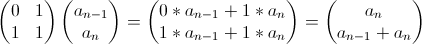Does the resultant matrix look familiar? If you look above, you’ll see that it’s the same as our recursive definition of the Fibonacci sequence! The top row is equal to the current number in the sequence (an) and the bottom row is equal to the next number in the sequence (an-1 + an = an+1). Continuing to multiply the resultant matrix by the Fibonacci matrix will cause consecutive entries to be produced. Because matrix multiplication is associative, we can move our multiplication to the exponent, and multiply that result by the first two terms in the sequence (0, 1), leading to our initial matrix:###References### 1: Strang, Gilbert. “Linear Algebra and Its Applications.”